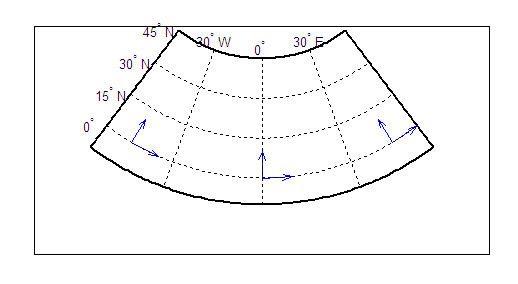# vfwdtran

Transform azimuth on ellipsoid to direction on map

## Syntax

```th = vfwdtran(lat,lon,az) th = vfwdtran(mstruct,lat,lon,az) [th,len] = vfwdtran(...) ```

## Description

`th = vfwdtran(lat,lon,az)` transforms the azimuth angle at specified latitude and longitude points on the sphere into the projection space. The map projection currently displayed is used to define the projection space. The input angles must be in the same units as specified by the current map projection. The inputs can be scalars or matrices of the equal size. The angle in the projection space is defined as positive counterclockwise from the x-axis.

`th = vfwdtran(mstruct,lat,lon,az)` uses the map projection defined by the input `mstruct` to compute the map projection.

`[th,len] = vfwdtran(...)` also returns the vector length in the projected coordinate system. A value of 1 indicates no scale distortion.

## Background

The direction of north is easy to define on the three-dimensional sphere, but more difficult on a two-dimensional map. For cylindrical projections in the normal aspect, north is always in the positive y-direction. For conic projections, north can be to the left or right of the y-axis. This function transforms any azimuth angle on the sphere to the corresponding angle in the projected paper coordinates.

## Examples

Sample calculations:

```axesm('eqdconic','maplatlim',[-10 45],'maplonlim',[-55 55]) gridm; framem; mlabel; plabel quiverm([0 0 0],[-45 0 45],[0 0 0],[10 10 10],0) quiverm([0 0 0],[-45 0 45],[10 10 10],[0 0 0],0)``````vfwdtran([0 0 0],[-45 0 45],[0 0 0]) ans = 59.614 90 120.39 vfwdtran([0 0 0],[-45 0 45],[90 90 90]) ans = -30.385 0.0001931 30.386 ```

## Limitations

This transformation is limited to the region specified by the frame limits in the current map definition.

## Tips

The geographic azimuth angle is measured clockwise from north. The projection space angle is measured counterclockwise from the x-axis.

This function uses a finite difference technique. The geographic coordinates are perturbed slightly in different directions and projected. A small amount of error is introduced by numerical computation of derivatives and the variation of map distortion parameters.

## Version History

Introduced before R2006a